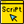# Paillier Cryptosystem in Python

Paillier public key cryptography with homomorphic propretiesSun 18 Apr 2021

•/Scripts

Paillier cryptosystem is a probabilistic asymmetric algorithm for public key cryptography and it is particularly interesting as it offers additive homomorphism.

#### And what is additive homomorphism?

It simply means that you can add together encrypted output and when you decrypt the result it will be the addition of the decrypted inputs.
In this article we are not going to talk about the mathematics behind the Paillier cryptosystem, which you can find also in the Wikipedia page, but the main point is that it is based on the difficulty to solve the problem of computing n-th residue classes.

#### What are the applications of an additive homomorphic system?

There are basically two main applications of Paillier that is particularly useful:

• Electronic Voting
• Electronic Cash

For example in electronic voting we can use it so people can vote anonymously, make sure that they have voted only once and that the vote is among the valid ones. If you would like to learn more about how Paillier works and why it works the way it does you can find a lot of information simply by googling about it, so I will not expand any further here.

The following implementation is in Python and with a key of 2048 bits using gmpy2. The use of gmpy2 is drastically improving the performance versus a pure python implementation.

``````import math
import random
from random import shuffle
import sys
import gmpy2
from time import time
from Crypto.Util.number import getPrime

# In:

def gcd(a,b):
while b > 0:
a, b = b, a % b
return a

def lcm(a, b):
return a * b // gcd(a, b)

def int_time():
return int(round(time() * 1000))

class PrivateKey(object):
def __init__(self, p, q, n):
#self.l = lcm(p-1,q-1)----This is added as requested by the setup BUT not used, shortcut is used!
self.l = (p-1) * (q-1)
#self.m = gmpy2.invert(gmpy2.f_div(gmpy2.sub(gmpy2.powmod(n+1,self.l,n*n),gmpy2.mpz(1)),pub.n),n) --- Shortcut used instead of it
self.m = gmpy2.invert(self.l, n)  #1/fi(n)
def __repr__(self):
return '<PrivateKey: %s %s>' % (self.l, self.m)

class PublicKey(object):

@classmethod
def from_n(cls, n):
return cls(n)
def __init__(self, n):
self.n = n
self.n_sq = n * n
self.g = n + 1
def __repr__(self):
return '<PublicKey: %s>' % self.n

def generate_keypair(bits):
p_equal_q = True
while p_equal_q:
p = getPrime(bits // 2)
q = getPrime(bits // 2)
if (p!=q):
p_equal_q = False
n = p * q
return PrivateKey(p, q, n), PublicKey(n)

def encrypt(pub, plain):
one = gmpy2.mpz(1)
state = gmpy2.random_state(int_time())
r = gmpy2.mpz_random(state,pub.n)
while gmpy2.gcd(r,pub.n) != one:
state = gmpy2.random_state(int_time())
r = gmpy2.mpz_random(state,pub.n)
x = gmpy2.powmod(r,pub.n,pub.n_sq)
cipher = gmpy2.f_mod(gmpy2.mul(gmpy2.powmod(pub.g,plain,pub.n_sq),x),pub.n_sq)
return cipher

def decrypt(priv, pub, cipher):
one = gmpy2.mpz(1)
x = gmpy2.sub(gmpy2.powmod(cipher,priv.l,pub.n_sq),one)
plain = gmpy2.f_mod(gmpy2.mul(gmpy2.f_div(x,pub.n),priv.m),pub.n)
if plain >= gmpy2.f_div(pub.n,2):
plain = plain - pub.n
return plain